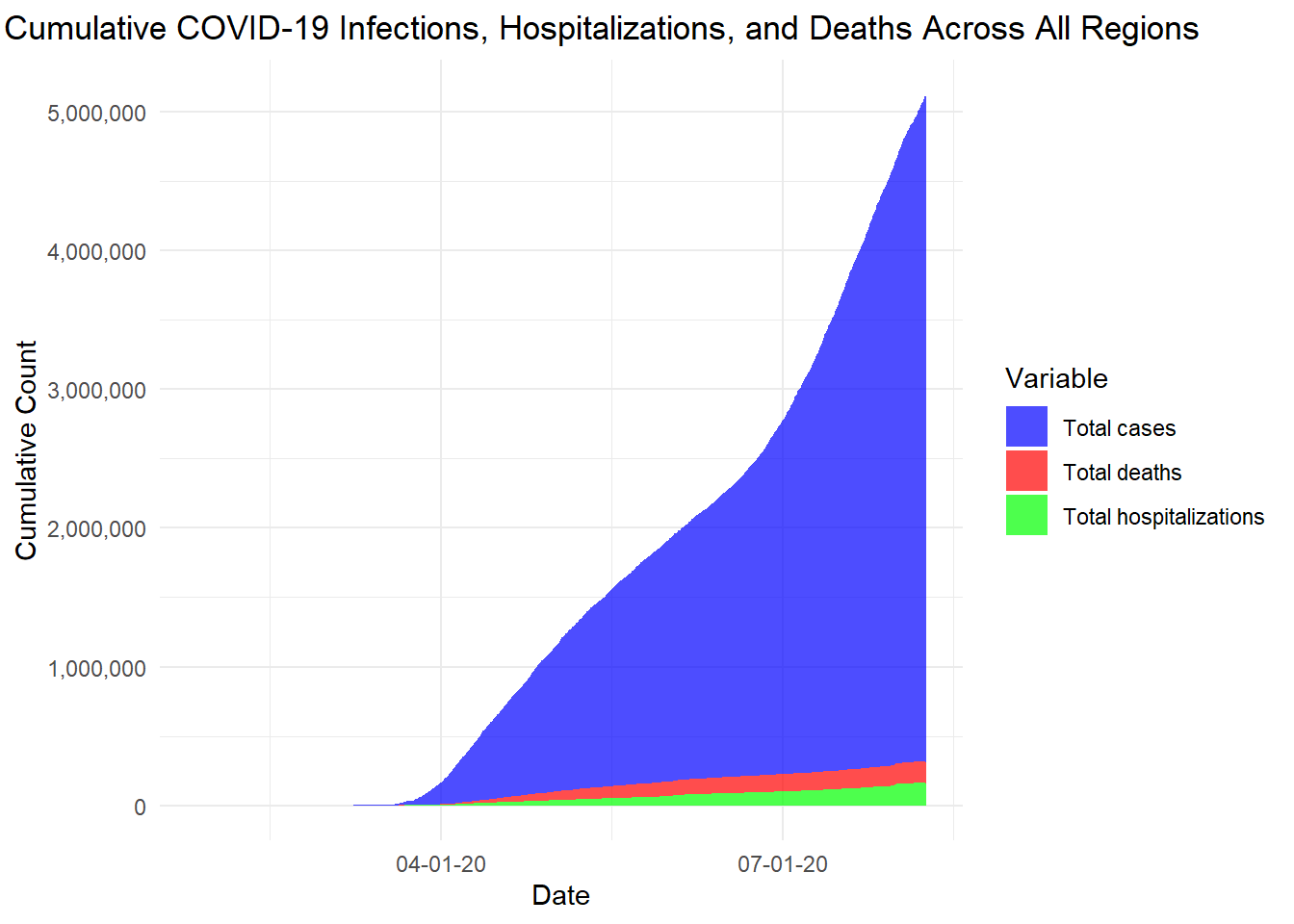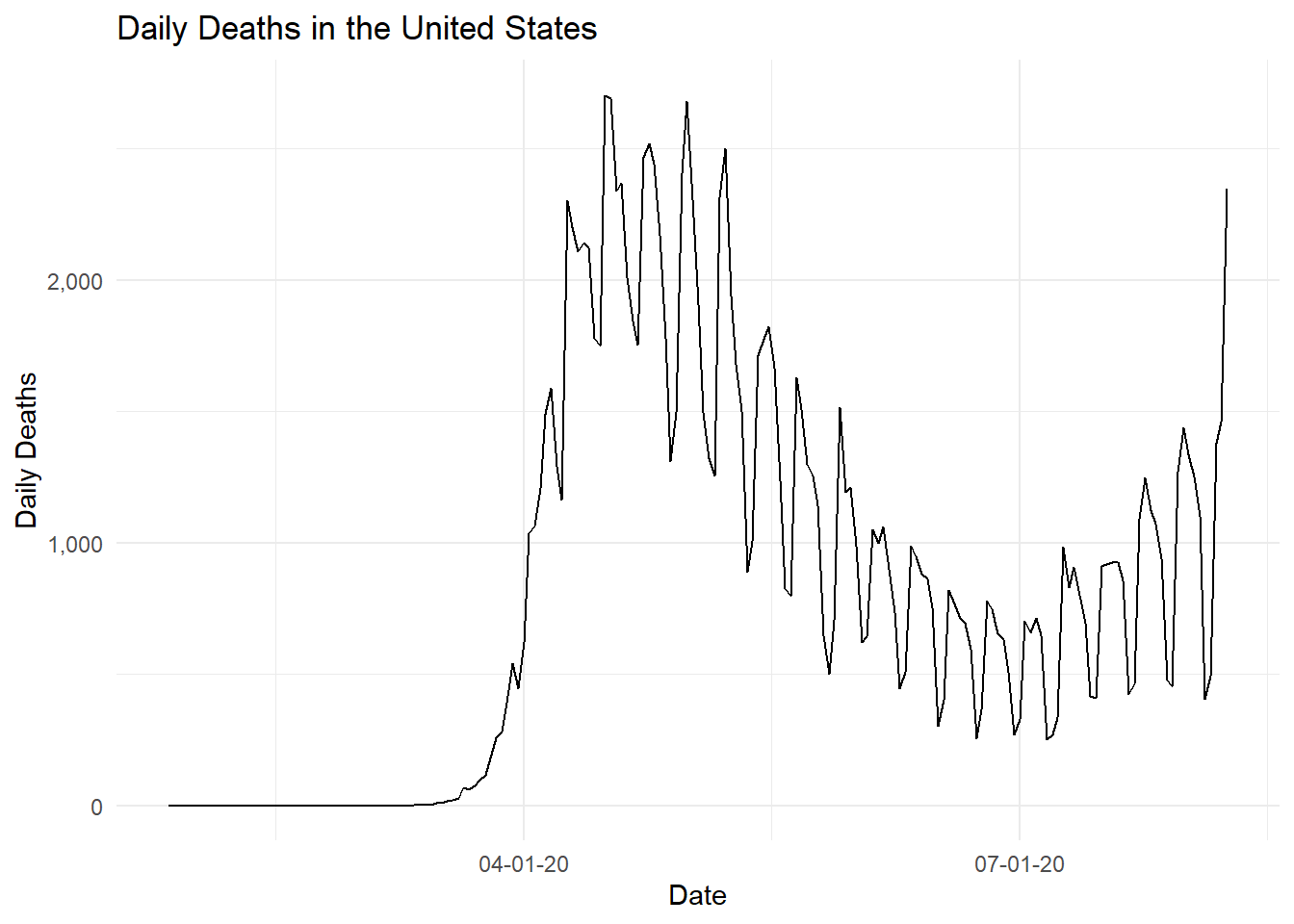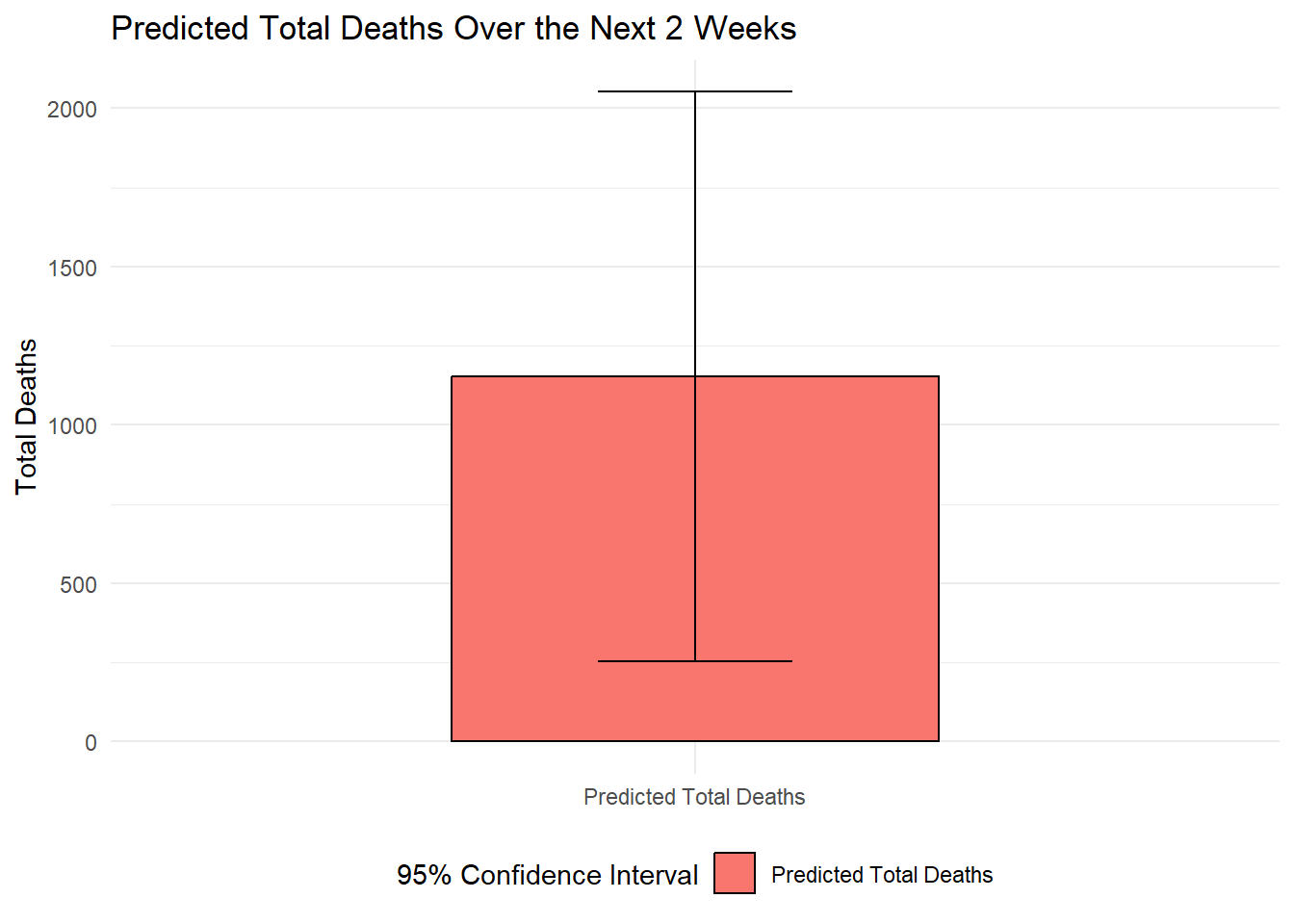# Section 1: Examining and cleaning the data

library(ggplot2)
library(reshape2)
library(dplyr)
library(lubridate)
library(zoo)
library(scales)
library(crayon)
library(tidyr)
1. Reading in the .csv. To replicate this R script, please change file path/working directory accordingly.
df <- read.csv("~/Old or unsorted/_covid_data_cases_deaths_hosp.csv")
1. Exploring and cleaning the data

First, examining the head of the data

head(df)
##   location_id Province.State           Country.Region  Date Confirmed Deaths
## 1         102                United States of America 43885        36      0
## 2         102                United States of America 43886        36      0
## 3         102                United States of America 43887        42      0
## 4         102                United States of America 43888        42      0
## 5         102                United States of America 43889        44      0
## 6         102                United States of America 43890        44      0
##   population Hospitalizations US.STATE
## 1  327978730               NA        1
## 2  327978730               NA        1
## 3  327978730               NA        1
## 4  327978730               NA        1
## 5  327978730               NA        1
## 6  327978730               NA        1

It looks like there are lots of “NA” and blank values. I will switch these to “0” to make aggregation easier.

df$Confirmed[is.na(df$Confirmed)] <- "0"

df$Hospitalizations[is.na(df$Hospitalizations)] <- "0"

df$Deaths[is.na(df$Deaths)] <- "0"

Making sure everything is the class I want

df$Confirmed <- as.numeric(df$Confirmed)
df$Hospitalizations <- as.numeric(df$Hospitalizations)
df$Deaths <- as.numeric(df$Deaths)

df$Date <- as.Date(df$Date)

df$Date <- df$Date - lubridate::years(70)

Fixing the blank state problem by replacing with “Unknown”

df$Province.State <- ifelse(is.na(df$Province.State) | df$Province.State == "", "Unknown", df$Province.State)

Fixing the missing dates problem, where not every state reports on every day. I also replace missing values with highest earlier reported value

imputed_data <- df %>%
group_by(Province.State) %>%
complete(Date = seq(min(Date), max(Date), by = "day")) %>%
arrange(Province.State, Date) %>%
fill(Confirmed, Deaths, Hospitalizations) %>%
mutate(
Confirmed = ifelse(Confirmed == 0 & lag(Confirmed, default = 1) > 0, lag(Confirmed), Confirmed),
Deaths = ifelse(Deaths == 0 & lag(Deaths, default = 1) > 0, lag(Deaths), Deaths),
Hospitalizations = ifelse(Hospitalizations == 0 & lag(Hospitalizations, default = 1) > 0, lag(Hospitalizations), Hospitalizations)
) %>%
ungroup()

imputed_data <- df %>%
group_by(Province.State) %>%
complete(Date = seq(min(Date), max(Date), by = "day")) %>%
arrange(Province.State, Date) %>%
fill(Confirmed, Deaths, Hospitalizations) %>%
mutate(
Confirmed = ifelse(Confirmed == 0, cummax(Confirmed), Confirmed),
Deaths = ifelse(Deaths == 0, cummax(Deaths), Deaths),
Hospitalizations = ifelse(Hospitalizations == 0, cummax(Hospitalizations), Hospitalizations)
) %>%
ungroup() 

# Section 2: Graphing aggregate cases, hospitalizations, and deaths over time

Aggregating all the data over all locations.

agg_data <- imputed_data %>%
group_by(Date) %>%
summarise(TotalConfirmed = sum(Confirmed),
TotalDeaths = sum(Deaths),
TotalHospitalizations = sum(Hospitalizations))

Plot 1 uses melt to make a stacked area graph

# Melt data
melted_data <- melt(agg_data, id.vars = "Date", measure.vars = c("TotalConfirmed", "TotalDeaths", "TotalHospitalizations"))

# Generate and display plot
ggplot(melted_data, aes(x = Date, y = value, fill = variable)) +
geom_area(alpha = 0.7) +
labs(title = "Total Cumulative COVID-19 Infections, Hospitalizations, and Deaths Across All Regions",
x = "Date",
y = "Cumulative Count",
fill = "Variable") +
theme_minimal() +
theme(plot.title = element_text(hjust = 0.5, lineheight = 1.2)) +
scale_fill_manual(values = c("TotalConfirmed" = "blue", "TotalDeaths" = "red", "TotalHospitalizations" = "green"),
labels = c("Total cases", "Total deaths", "Total hospitalizations")) +
scale_y_continuous(labels = scales::comma) +  # Display y-axis labels with commas
scale_x_date(labels = date_format("%m-%d-%y"))# Section 3: Graphing the daily death curve

The way I structured this code is to first generate the change in the cumulative number of deaths in each state, with 0 as the lower bound. Then, I aggregate that “daily deaths per state” figure over all states, and plot.

Calculating daily deaths per state

imputed_data <- imputed_data %>%
group_by(Province.State) %>%
mutate(dailyDeaths = Deaths - lag(Deaths, default = 0)) %>%
mutate(dailyDeaths = ifelse(dailyDeaths < 0, 0, dailyDeaths))

Aggregating daily deaths across all states

agg_data <- imputed_data %>%
group_by(Date) %>%
summarise(TotalDailyDeaths = sum(dailyDeaths))

Creating the plot

ggplot(agg_data, aes(x = Date, y = TotalDailyDeaths)) +
geom_line() +
labs(title = "Daily Deaths in the United States",
x = "Date",
y = "Daily Deaths") +
theme_minimal() +
scale_y_continuous(labels = scales::comma) +
scale_x_date(labels = date_format("%m-%d-%y"))# Section 4: Predicting daily deaths in the next 2 weeks

I build heavily upon my work in part 2. I generate 1) a new variable which is equal to the total deaths in the last 2 weeks for each date, then the average of that 2) a new variable which is equal to deaths in the next 2 weeks for each date, then the average of that 3) then create a linear regression model in which the outcome is the average of the next 2 weeks and the exposure is the average of the previous 2 weeks.

Calculating the sum of daily deaths over the previous and next 2 weeks

agg_data <- agg_data %>%
mutate(Prev2Weeks = rollapply(TotalDailyDeaths, width = 15, FUN = sum, fill = NA, align = "right"),
Next2Weeks = (rollapply(TotalDailyDeaths, width = 15, FUN = sum, fill = NA, align = "left") - TotalDailyDeaths))

Calculating the average daily deaths over the last 2 weeks and next 2 weeks

agg_data <- agg_data %>%
mutate(AvgLast2Weeks = ((Prev2Weeks + TotalDailyDeaths) / 15),
AvgNext2Weeks = ( Next2Weeks/14))

Building a linear model with AvgNext2Weeks as the outcome and AvgLast2Weeks as the predictor

model <- lm(AvgNext2Weeks ~ AvgLast2Weeks, data = agg_data)

Predicting the average daily deaths over the next 2 weeks after the last observed date

last_observed_date <- max(agg_data$Date) last_observed_date <- as.Date(last_observed_date, origin = "1970-01-01") new_avg_last_2_weeks <- agg_data$AvgLast2Weeks[which.max(agg_data\$Date == last_observed_date)]
predicted_avg_next_2_weeks <- predict(model, newdata = data.frame(AvgLast2Weeks = new_avg_last_2_weeks), interval = "prediction", level = 0.95)

Calculating the predicted total deaths over the next 2 weeks

predicted_total_deaths_next_2_weeks <- sum(predicted_avg_next_2_weeks[, 1])

Calculating the lower and upper bounds of the confidence intervals

lower_bound <- sum(predicted_avg_next_2_weeks[, 2])
upper_bound <- sum(predicted_avg_next_2_weeks[, 3])

Printing the predicted total deaths and confidence intervals over the next 2 weeks

cat("Predicted Total Deaths Over the Next 2 Weeks:", predicted_total_deaths_next_2_weeks, "\n")
## Predicted Total Deaths Over the Next 2 Weeks: 1152.884
cat("95% Confidence Interval (Lower Bound):", lower_bound, "\n")
## 95% Confidence Interval (Lower Bound): 254.1438
cat("95% Confidence Interval (Upper Bound):", upper_bound, "\n")
## 95% Confidence Interval (Upper Bound): 2051.624

Creating the visualization data

visualization_data <- data.frame(
Label = c("Predicted Total Deaths"),
Deaths = c(predicted_total_deaths_next_2_weeks),
LowerCI = c(lower_bound),
UpperCI = c(upper_bound)
)

Bar plot with error bars

ggplot(visualization_data, aes(x = Label, y = Deaths, fill = Label)) +
geom_bar(stat = "identity", width = 0.5, color = "black") +
geom_errorbar(aes(ymin = LowerCI, ymax = UpperCI), width = 0.2, color = "black",
position = position_dodge(0.5)) +
theme_minimal() +
labs(title = "Predicted Total Deaths Over the Next 2 Weeks",
y = "Total Deaths",
x = NULL) +
theme(legend.position = "bottom") +
guides(fill = guide_legend(title = "95% Confidence Interval"), override.aes = list(fill = "white"))